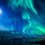# Synthetic Geometry Group - Karthik's Proposal

Here are my submissions :

1) Let $\Delta ABC$ have cevians $\overline{AD}$ and $\overline{CE}$, which meet at a point $F$ inside the triangle. Prove that $[\Delta ABC] \cdot [\Delta DEF] = [\Delta BDE] \cdot [\Delta AFC]$, where $[ A ]$ denotes area of figure $A$.

2) If a cevian $\overline{AQ}$ of an equilateral triangle $ABC$ is extended to meet the circumcircle at $P$, prove that $\dfrac{1}{\overline{PB}} + \dfrac{1}{\overline{PC}} = \dfrac{1}{\overline{PQ}}$.Note by Karthik Venkata
4 years, 2 months ago

This discussion board is a place to discuss our Daily Challenges and the math and science related to those challenges. Explanations are more than just a solution — they should explain the steps and thinking strategies that you used to obtain the solution. Comments should further the discussion of math and science.

When posting on Brilliant:

• Use the emojis to react to an explanation, whether you're congratulating a job well done , or just really confused .
• Ask specific questions about the challenge or the steps in somebody's explanation. Well-posed questions can add a lot to the discussion, but posting "I don't understand!" doesn't help anyone.
• Try to contribute something new to the discussion, whether it is an extension, generalization or other idea related to the challenge.

MarkdownAppears as
*italics* or _italics_ italics
**bold** or __bold__ bold
- bulleted- list
• bulleted
• list
1. numbered2. list
1. numbered
2. list
Note: you must add a full line of space before and after lists for them to show up correctly
paragraph 1paragraph 2

paragraph 1

paragraph 2

[example link](https://brilliant.org)example link
> This is a quote
This is a quote
    # I indented these lines
# 4 spaces, and now they show
# up as a code block.

print "hello world"
# I indented these lines
# 4 spaces, and now they show
# up as a code block.

print "hello world"
MathAppears as
Remember to wrap math in $$ ... $$ or $ ... $ to ensure proper formatting.
2 \times 3 $2 \times 3$
2^{34} $2^{34}$
a_{i-1} $a_{i-1}$
\frac{2}{3} $\frac{2}{3}$
\sqrt{2} $\sqrt{2}$
\sum_{i=1}^3 $\sum_{i=1}^3$
\sin \theta $\sin \theta$
\boxed{123} $\boxed{123}$

Sort by:

Question 1: Extend segment BF to hit AC at T. Construct $DE$ and call its intersection point with $BE$ , $M$. Notice that the desired relation is equivalent to $\frac{[DEF]}{[BDE]} = \frac{[AFC]}{[ABC]} \implies \frac{FM}{MB} = \frac{TF}{TB}$. We will use mass points. Put weights of $p, q, r$ on $A, B, C$ respectively. This implies that $T$ has a mass of $p+r$ with implies that $\frac{TF}{BF} = \frac{q}{p+r} \implies \frac{TF}{TB} = \frac{q}{p+q+r}$

Notice that $F$ has mass $p+q+r$. This implies that $\frac{MF}{MB} = \frac{q}{p+q+r}$ and we are done.

- 4 years, 2 months ago

Nice use of Barycentric Coordinates !

- 4 years, 2 months ago

Question 2: We know by Ptomely's, that $AP = PB + PC$.

Then we just need to prove that $\frac{1}{PB} + \frac{1}{PC} = \frac{AP}{PB \cdot PC} = \frac{1}{PQ} \implies \frac{BP}{AP} = \frac{PQ}{CP}$

Observe that $\angle ABP = \angle ACB = 60^{\circ}$ and $\angle APC = \angle ABC = 60^{\circ}$ because they substend the same arc. Similarly, $\angle CBP = \angle CAP$ substend the same arc. This implies that $\triangle BPQ \sim APC$ and the desired ratio is given with these proportional lengths.

- 4 years, 2 months ago

@Alan Yan Do check problem no. 5 in RMO board-2 by Nihar Mahajan ! It's more interesting than these :). Link

- 4 years, 2 months ago

@Xuming Liang @Calvin Lin @Sualeh Asif Here are some simple problems ! Presently not very good at Geometry, but planning to improve.

- 4 years, 2 months ago